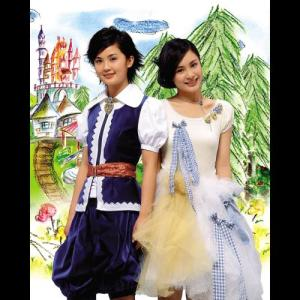# How Much

Twins2004年8月26日

## How Much 歌詞

How much is that pencil in the window

A dollar is all it will be

A pencil is missing from my school bag

A pencil is all that i need

How much Oh how much

How much will these things all cost me

How much oh how much

How much will these things all cost me

How much is that rubber in the window

Two dollars is all it will be

A rubber is missing from my school bag

A rubber is all that I need

How much Oh how much

How much will these things all cost me

How much oh how much

How much will these things all cost me

How much is that ruler in the window

Three dollars is all it will be

A ruler is missing from my school bag

A ruler is all that I need

How much Oh how much

How much will these things all cost me

How much oh how much

How much will these things all cost me

How much is that notebook in the window

Four dollars is all it will be

A notebook is missing from my school bog

A notebook is all that I need

How much Oh how much

How much will these things all cost me

How much oh how much

How much will these things all cost me